# INDEX and MATCH

An alternative to using the VLOOKUP function is to combine the INDEX and MATCH functions.
The VLOOKUP function can only lookup a value in the first column.
Using this combination you can lookup a value in ANY column.
This combination can be used as an alternative to using the VLOOKUP (and HLOOKUP) functions.
This combination should be used instead of the OFFSET and MATCH combination.

### Understanding the INDEX and MATCH Combination

This lets you lookup a value in ANY column of a table and returns a value from any column.
The MATCH function can be used to find the position of a value in a single row or single column.
This is used to find the position (or row number) of the value you are looking up in the lookup column.
The INDEX function can be used to return the value in the same row but in a different column.

### Return the Exact Match (vlookup equivalent)

The MATCH function will look at the list in cells B2:B14 and return the position for the string "Japan" (which is 4).
The INDEX function in the first example returns the 4th item from the list in cells C2:C14 (which is 56).
The INDEX function in the second example returns the 4th item from the list in cells D2:D14 (which is Tokyo).
The table does not have to be sorted when looking for an exact match.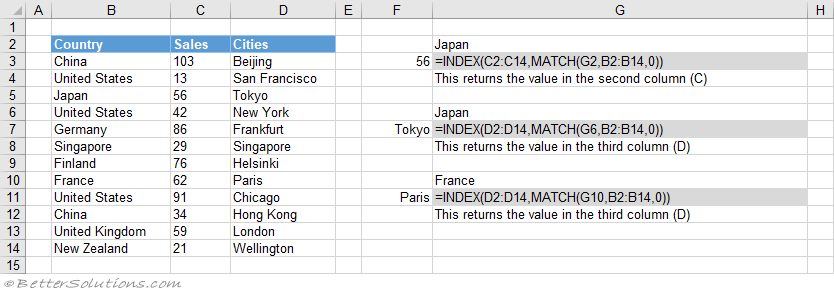### Return the Exact Match (hlookup equivalent)

An alternative to you the HLOOKUP function is to use the INDEX and MATCH functions.
The table does not have to be sorted when looking for an exact match.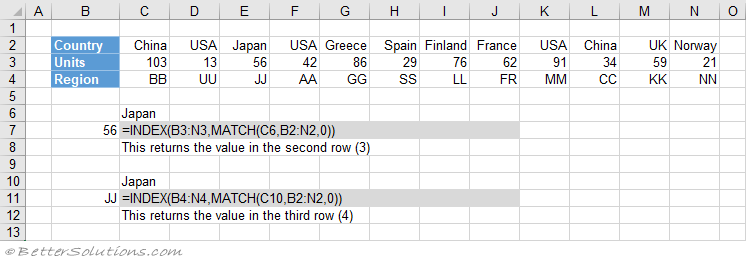### Return the Exact Match (not the first column)

When the "match_type" argument is 0 an exact match is found.
If there is more than one occurrence, the first occurrence is used.
The definition of the first occurrence is the same regardless of whether the table is sorted.
The table does not have to be sorted when looking for an exact match.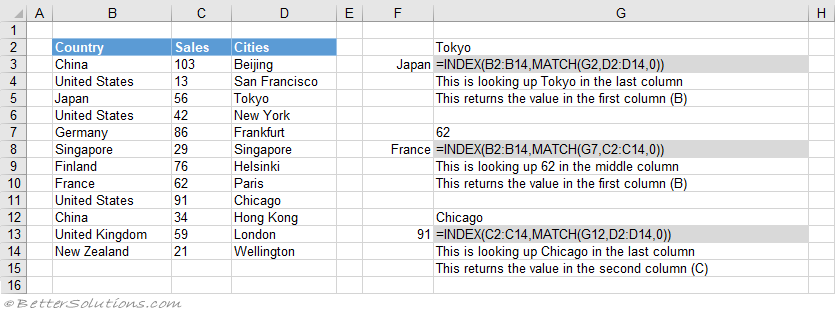### Return the Exact Match (item does not exist)

When the MATCH "lookup_value" argument is not in the list, #N/A is returned.
When the "match_type" argument is 0 an exact match is found
The table does not have to be sorted when looking for an exact match.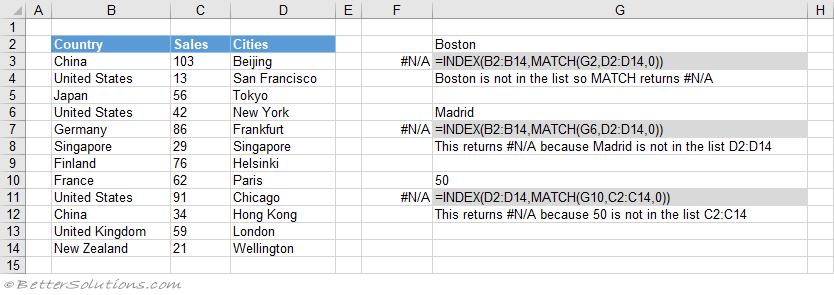### Return the Exact Match (case sensitive)

This combination is not case sensitive because the MATCH function is not case sensitive.
This means that upper case and lower case characters are equal (for example Japan = JAPAN)
You can perform a case sensitive lookup by using the EXACT function.
When the "match_type" argument is 0 an exact match is found.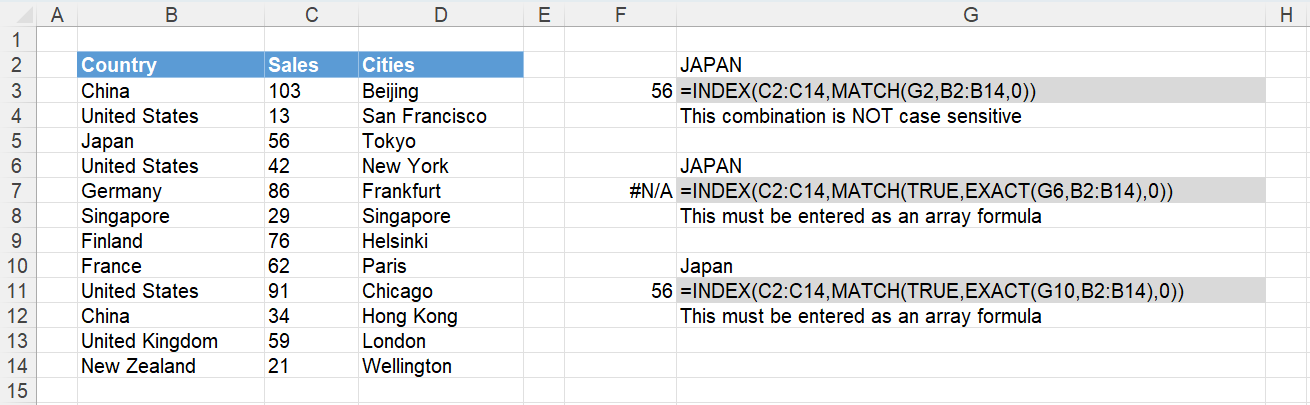### Return the Exact Match (first occurrence, sorted or not sorted)

This combination will always return the first occurrence, starting at the top.
When the "match_type" argument is 0 an exact match is found.
This table does not have to be sorted to return the first occurrence.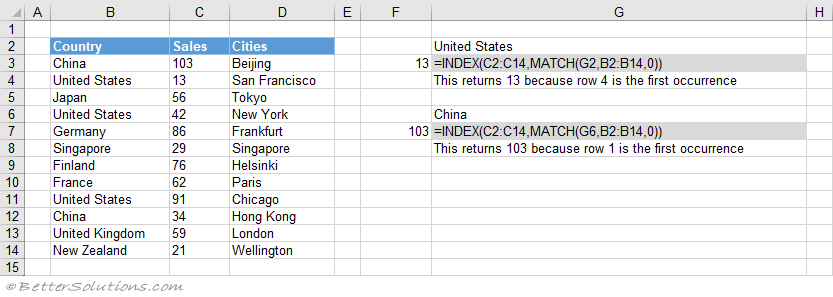### Return the Exact Match (last occurrence, sorted)

This can achieved by using the COUNTIF function.
The MATCH function will return the first occurrence which is then added to the total number of occurrences to return the position of the last occurrence.
The table has been sorted by Sales and then by Country in ascending order.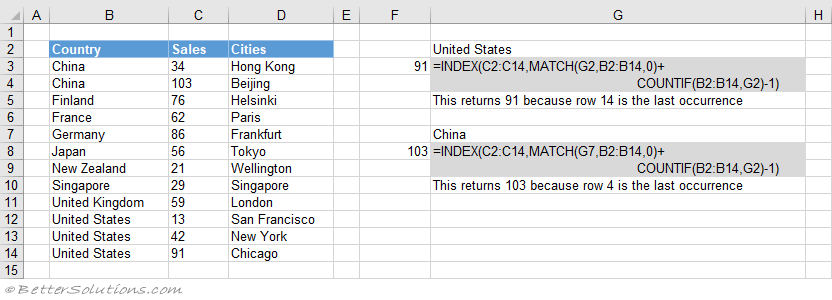### Return the Exact Match (last occurrence, not sorted)

The last occurrence can be achieved when the "lookup_value" column is not sorted.
The array eventually contains either 1's or error values and because the "lookup_value" is 2 it will return the position of the last 1 in the array.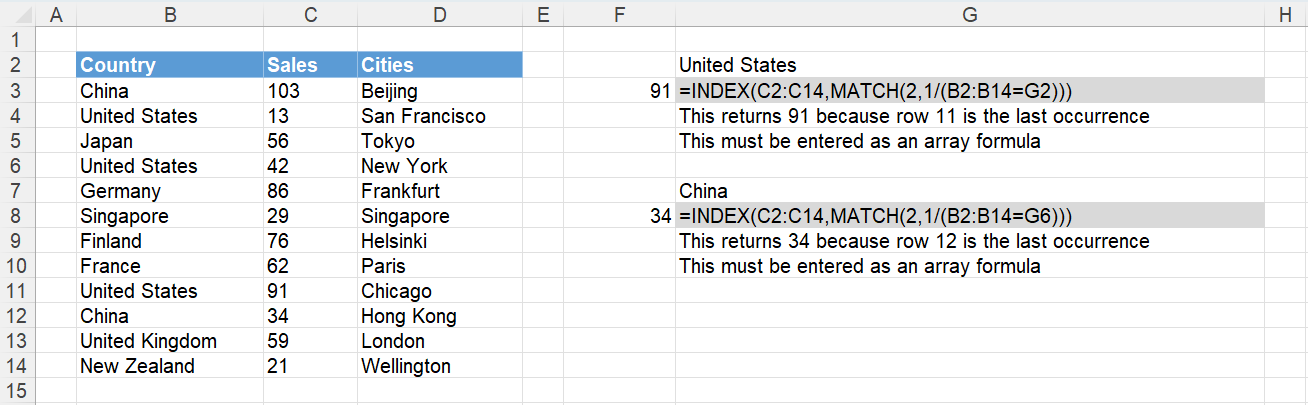### Return the Exact Match (with wildcard *)

An asterisk (*) matches any sequence of characters.
The MATCH function does support wildcard characters.
The "match_type" argument must be 0.
You can search for the asterisk character by prefixing it with a tilde?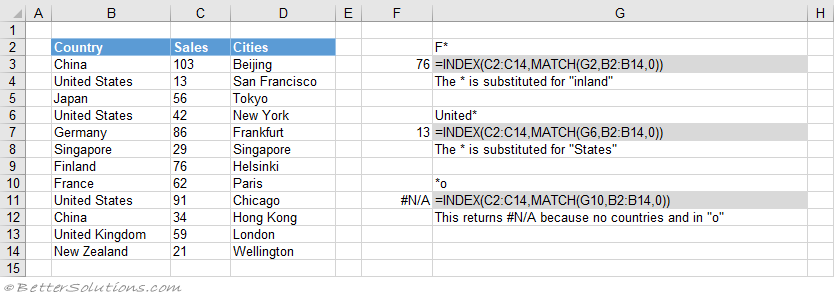### Return the Exact Match (with wildcard ?)

A question mark (?) matches any single character.
The MATCH function does support wildcard characters.
When the "match_type" argument is 0 an exact match is found.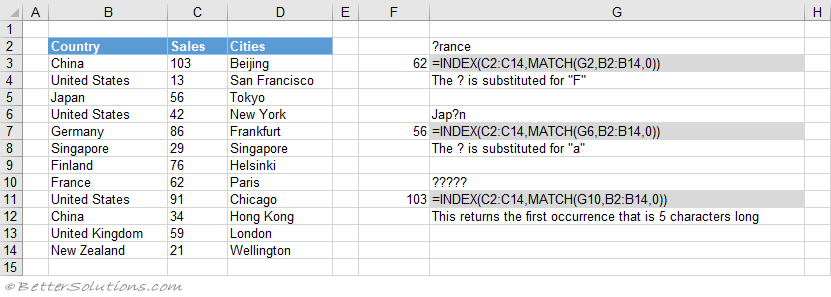### Return the Exact Match (multiple criteria)

When you want to return a value in the same row after finding matching values in 2 (or more) columns.

### Return the Closest Match (next smallest)

You can get the next smallest value when the "lookup_value" column is sorted into Ascending Order.
When the "match_type" argument is 1 the next smallest value is found.
The table has been sorted by Sales into ascending order.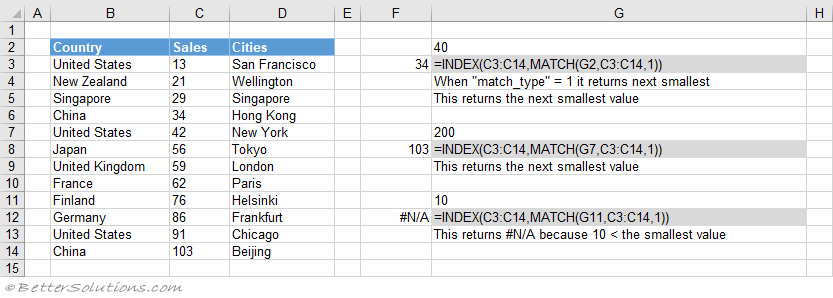### Return the Closest Match (next largest)

You can get the next largest value when the "lookup_value" column is sorted into Descending Order.
When the "match_type" argument is -1 the next largest value is found.
The table has been sorted by Sales into descending order.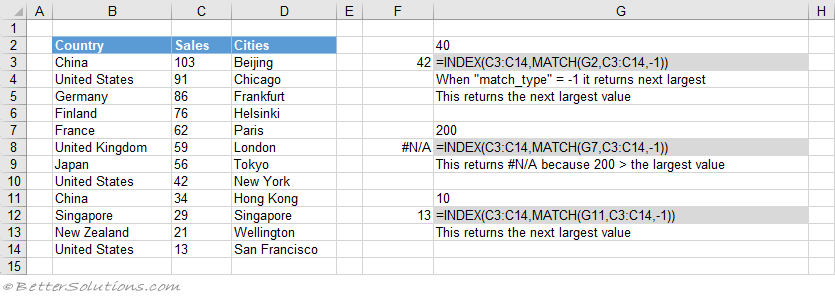### Important

If you have trailing spaces in your data then you might want to add an asterisk wildcard to the end of the "lookup_value".
The Lookup Wizard provided an easy way to insert these types of formulas however it was removed in Excel 2010.